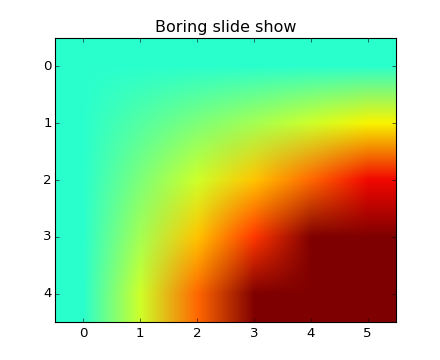### We're updating the default styles for Matplotlib 2.0

Learn what to expect in the new updates#### Previous topic

pylab_examples example code: anchored_artists.py

#### Next topic

pylab_examples example code: annotation_demo.py

# pylab_examples example code: animation_demo.py¶```"""
Pyplot animation example.

The method shown here is only for very simple, low-performance
use.  For more demanding applications, look at the animation
module and the examples that use it.
"""

import matplotlib.pyplot as plt
import numpy as np

x = np.arange(6)
y = np.arange(5)
z = x * y[:,np.newaxis]

for i in xrange(5):
if i==0:
p = plt.imshow(z)
fig = plt.gcf()
plt.clim()   # clamp the color limits
plt.title("Boring slide show")
else:
z = z + 2
p.set_data(z)

print("step", i)
plt.pause(0.5)
```

Keywords: python, matplotlib, pylab, example, codex (see Search examples)